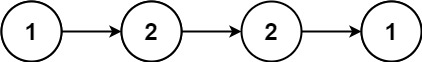# Leetcode 234 - Palindrome linked list

Note:

• For O(1) space solution, we need to reverse second half linked list then use double pointers.
• How to reverse the second half list? Fast and slow pointers.
• Because fast moves twice faster than slow. If the length is odd, fast will be at the end. If the length is even, fast will be one node away from the end.
• We make sure that the first half is at least as long as the second half, so it needs to keep the middle node when the length is odd.
• After reversing the second half, it’s detached from the first half because now its end is null instead of the middle node.
• Use double pointers to iterate. We stop when we hit the end of second half because sometimes the first half can have one more node than the second half.

Question:

Given the head of a singly linked list, return true if it is a palindrome.

Example:Code:
Double pointers - O(1) space

Double pointers with O(n) space